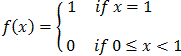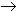#Interactive Real Analysis

Next | Previous | Glossary | Map

## 8.1. Pointwise Convergence

### Example 8.1.7 (b): Pointwise Convergent Function Sequence

Show that fn(x) = xn, x[ 0, 1 ] converges pointwise and identify the limit function.

 LetThen: if x = 1 we have fn(1) = 1 for all n if x < 1 then fn(x) = xn is the power sequence and thus converges to zero Hence fn(x)f(x) for each fixed x.
Next | Previous | Glossary | Map